# The steel pipe AB has a 102-mm outer diameter and a 6-mm wall thickness. Knowing that arm CD is rigidly attached to the pipe, determine the principal stresses and die maximum shearing stress at point K.

Question-AnswerCategory: Strength of MaterialsThe steel pipe AB has a 102-mm outer diameter and a 6-mm wall thickness. Knowing that arm CD is rigidly attached to the pipe, determine the principal stresses and die maximum shearing stress at point K.

The steel pipe AB has a 102-mm outer diameter and a 6-mm wall thickness. Knowing that arm CD is rigidly attached to the pipe, determine the principal stresses and die maximum shearing stress at point K.Step No: 1

Use the following relation to calculate the inner diameter of the Steel pipe: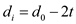Here, the outer diameter isand thickness of the pipe is t.
Substitute 102 mm forand 6 mm for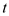.Use the following relation to calculate the polar moment of inertia of the Steel pipe:Substitute 102 mm forand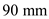for.Step No: 2

Calculate the moment of inertia of the Steel pipe.Substitute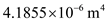for:Step No: 3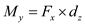Here,is the force in the x-direction andis the perpendicular distance.
Substitute 10000 N for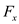and 200 mm for.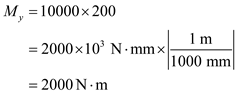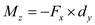Here,is the force in the x-direction and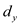is the perpendicular distance.
Substitute 10000 N forand 150 mm for.Here, the negative sign in the moment about z-direction is due to the moment acts in the clockwise direction.
The force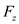creates the moment about z axis and the force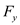creates the torsion in y direction. Therefore, the momentsand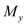cause for the bending and twisting moments respectively and the respective stresses are bending stress and torsional shear stress.

Step No: 4

Calculate the bending stress at K as follows:Here, distance of the point K from the axis is c.
Substitutefor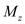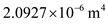forand 0.051 m for y.Here, the negative sign indicates that the stress at the point K is compressive in nature.

Step No: 5

Calculate the shear stress at K as follows:Substitute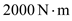forforand 0.051 m for r.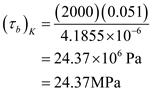Step No: 6

Calculate the average stress as follows: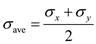Substitute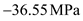forand 0 for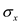Calculate the maximum shear stress as follows.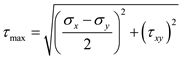Substitute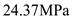forforand 0 for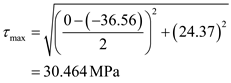Therefore, the maximum shear stress is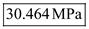.

Step No: 7

Calculate the maximum normal stress at K as follows.Substitutefor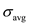andfor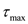.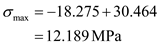Therefore, the maximum normal stress at K is.

Step No: 8

Calculate the minimum normal stress at K as follows.Substituteforandfor.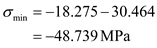Therefore, the minimum normal stress at K is.